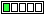All about flooble | fun stuff | Get a free chatterbox | Free JavaScript | Avatarsperplexus dot infoPolynomial and exponential (Posted on 2023-09-15)Determine all pairs of positive integers (x, y) such that x4 - 6x2 + 1 = 7·2y

 No Solution Yet Submitted by Danish Ahmed Khan No RatingComments: ( Back to comment list | You must be logged in to post comments.)solution| Comment 2 of 3 |let z = x^2, then

z^2 - 6z + 1 = 7*2^y

z^2 - 6z + 8 = 7*(2^y + 1)

(z - 4)(z - 2) = 7*(2^y + 1)

Now the RHS is odd because it's the product of two odd factors, so z must also be odd. And since z is also a square, z = 1 mod 8.

The LHS mod 8 then is (1 - 4)(1 - 2) = 3 and so the RHS must also = 3 mod 8. Well, 7 = -1 mod 8 so (2^y + 1) = -3 mod 8 = 5 mod 8, and that means 2^y = 4 mod 8

But 2^y is a multiple of 8 whenever y >= 3, and so the only way 2^y = 4 mod 8 is if 2^y = 4 and y= 2

If y = 2, then the RHS = 7*(2^2 + 1) = 7*5 = 35

Since prime decompositions are unique, it must be that z-2 and z-4 are also 7 and 5, which requires that z = 9. Finally, since z = x^2 and x is positive, x = 3

The only solution in positive integers, then, is (x,y) = (3,2)

 Posted by Paul on 2023-09-15 15:12:32Please log in:

 Search: Search body:
Forums (0)